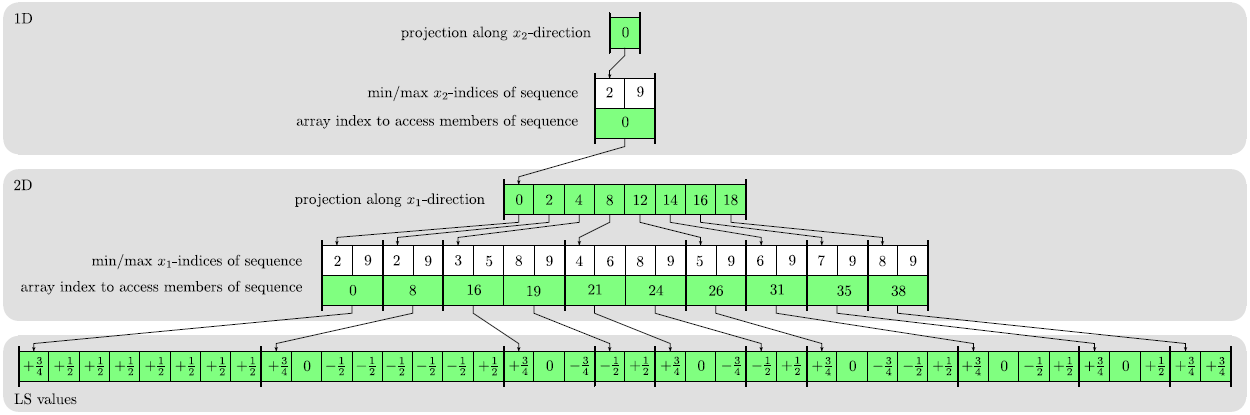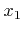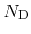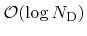Next: 3.5.4 Hierarchical Run-Length Encoding Up: 3.5 Level Set Data Previous: 3.5.2 Run-Length Encoding

## 3.5.3 Dynamic Tubular Grid

Another data structure for storing only the LS values of defined grid points is the dynamic tubular grid (DTG) . The DTG is hierarchically organized over the dimensions and is able to store a certain subset of grid points together with some data. The DTG can be used to store sparse data on grids with an arbitrary number of dimensions. The basic structure of a DTG is shown in Figure 3.5.The basic idea of the DTG is to apply subsequent orthogonal projections along all grid directions on the set of defined grid points. First, a projection along the-direction leads to a set of defined grid points in a lower-dimensional subspace, which is stored using a DTG with lower dimensionality. Hence, the DTG is recursively defined. For each projected defined grid point an index is stored in this lower-dimensional DTG. This index gives access to all the defined grid points which are projected to the same lower-dimensional grid point. Sequences of defined grid points along the projection direction are stored in a compact way using the minimum and maximum indices and an index giving access to the individual members of the sequence.

The memory requirements of the DTG scale linearly with the number of defined grid points,. Random access to the data of grid points requires a binary search within the array holding the minimum and maximum indices of sequences along each grid direction. Similarly to the RLE data structure, the binary searches lead to a worst case logarithmic complexity. In practice, since the data structure is very cache coherent, random access is almost as fast as for a full grid.

Sequential access can be realized in constant time using iterators moving over the data structure in lexicographical order as described in . Neighbor access to grid points, which is essential in calculating finite differences, can also be performed in constant time, if a stencil of iterators is moved simultaneously over the data structure.

The DTG allows storage of defined grid points on an infinite domain. The indices of points can take arbitrary values and do not need to be within a certain range, as is the case for a full grid representation. (The index values are only limited by the minimum and maximum possible representable values of the used integral data type.) For a full grid to mimic an infinite grid, grid extensions must be adapted, when the LS reaches the full grid boundary.Next: 3.5.4 Hierarchical Run-Length Encoding Up: 3.5 Level Set Data Previous: 3.5.2 Run-Length Encoding

Otmar Ertl: Numerical Methods for Topography Simulation